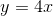# High School Math : Understanding Direct Proportionality

## Example Questions

### Example Question #1 : Understanding Direct Proportionality

Sarah notices her map has a scale of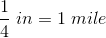.  She measures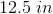between Beaver Falls and Chipmonk Cove.  How far apart are the cities?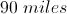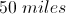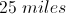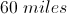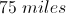Explanation:is the same as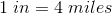So to find out the distance between the cities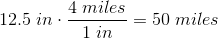### Example Question #2 : Understanding Direct Proportionality

Sunshine paint is made by mixing three parts yellow paint and one part red paint. How many gallons of yellow paint should be mixed with two quarts of red paint?

(1 gallon = 4 quarts)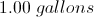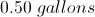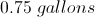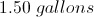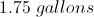Explanation:

First set up the proportion: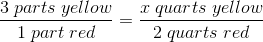x =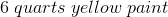Then convert this to gallons: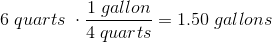### Example Question #3 : Understanding Direct Proportionality

Ifis directly proportional to, andwhen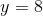, find a formula for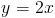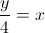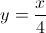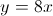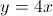Explanation: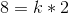sinceandSolving for, you get that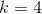Replacingin the original equation, you get the answer of